Test: Graph Theory - 2

# Test: Graph Theory - 2

Test Description

## 15 Questions MCQ Test Network Theory (Electric Circuits) | Test: Graph Theory - 2

Test: Graph Theory - 2 for Electrical Engineering (EE) 2023 is part of Network Theory (Electric Circuits) preparation. The Test: Graph Theory - 2 questions and answers have been prepared according to the Electrical Engineering (EE) exam syllabus.The Test: Graph Theory - 2 MCQs are made for Electrical Engineering (EE) 2023 Exam. Find important definitions, questions, notes, meanings, examples, exercises, MCQs and online tests for Test: Graph Theory - 2 below.
Solutions of Test: Graph Theory - 2 questions in English are available as part of our Network Theory (Electric Circuits) for Electrical Engineering (EE) & Test: Graph Theory - 2 solutions in Hindi for Network Theory (Electric Circuits) course. Download more important topics, notes, lectures and mock test series for Electrical Engineering (EE) Exam by signing up for free. Attempt Test: Graph Theory - 2 | 15 questions in 45 minutes | Mock test for Electrical Engineering (EE) preparation | Free important questions MCQ to study Network Theory (Electric Circuits) for Electrical Engineering (EE) Exam | Download free PDF with solutions
 1 Crore+ students have signed up on EduRev. Have you?
Test: Graph Theory - 2 - Question 1

### If [A] Matrix is Incidence matrix then which one of the following is true?

Detailed Solution for Test: Graph Theory - 2 - Question 1

Incidence matrix:

• It is the matrix that gives a relation between the branches and nodes.
• The rows of the incidence matrix [A] represent the number of nodes and the column of the matrix represents the number of branches in the given graph.
• If there are ‘n’ number of rows in a given incidence matrix[A], that means in a graph there are ‘n’ number of nodes.
• Similarly, if there are ‘b’ numbers of columns in that given incidence matrix[A], that means in that graph there are ‘b’ number of branches.
• We can construct the incidence matrix for the directed graph. We can draw a graph with the help of the incidence matrix.
• The algebraic sum of elements of all the columns is zero.
• The rank of the incidence matrix is (n–1).
• The determinant of the incidence matrix of a closed loop is zero.

Hence, an Only statement I is correct.

Test: Graph Theory - 2 - Question 2

### For a network graph having its fundamental loop matrix Bf and its sub-matrices Bt and Bl corresponding to twigs and links, which of the following statements are correct? 1) Bl is always an identity matrix. 2) Bt is an identity matrix. 3) Bf has rank of b – (n – 1), where b is the number of branches and n is the number of nodes of the graph.

Detailed Solution for Test: Graph Theory - 2 - Question 2

Fundamental loop: A fundamental loop is a closed path of a given graph with only one Link and the rest of them as twigs.

The number of fundamental loops for any given graph = b – (n – 1) = number of Links

These fundamental loop currents are called Tie set currents and the orientation of the tie set currents governed by the link.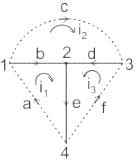Number of fundamental loops = 6 – 4 + 1 = 3

Fundamental loop 1 is a, b, e with b and e as twigs and a as Link. i1 is Tie set current and the direction as same as link ‘a’.

Similarly, loop2 → b, c, d → i2

loop3 → a, e, f → i3

Tie set matrix:

• It gives the relation between tie set currents and branch currents.
• The rows of a matrix represent the tie – set currents.
• The columns of a matrix represent branches of the graph.
• The order of the tie set matrix is (b – n + 1) × b
• A tie-set matrix can be assumed to be comprised of two submatrices.

Where, Bt and Bl are the sub-matrices of tie-set matrix (Bf) corresponding to twigs and links of a connected graph, respectively.

The elements of tie set matrix [M] = [aij]

[aij] = +1, If jth branch current is incident at ith tie set current at oriented in the same direction.

= –1, if jth branch current is incident at ith tie set current at oriented in the opposite direction.

= 0, If jth branch current is not incident with ith tie set current.

For the above graph, the tie set matrix is given by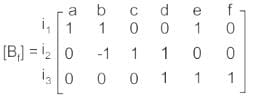We can rearrange the matrix as given below.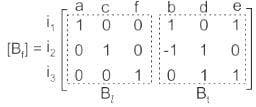Tie set currents and branch currents together form an identity matrix as marked in the above Tie set matrix.

Now, from the above matrix, it is clear that,

• The submatrix corresponding to twigs (Bt)­ is not an identity matrix
• The submatrix corresponding to twigs (Bl)­ is an identity matrix
• The rank of tie set matrix is b – (n – 1) i.e. 3 for the above matrix
Test: Graph Theory - 2 - Question 3

### Following is not the property of a complete incidence matrix:

Detailed Solution for Test: Graph Theory - 2 - Question 3

Incidence matrix:

• It is the matrix which gives relation between branches and nodes.
• The rows of matrix represent the number of nodes and the columns of matrix represents the numbers of branches.
• We can construct the incidence matrix for the directed graph. We can draw a graph with the help of incidence matrix.
• The algebraic sum of elements of all the columns is zero.
• The rank of incidence matrix is (n–1).
• The order of  incidence matrix will be (n × b).
• The determinant of the incidence matrix of a closed loop is zero.

The elements of incidence matrix are given by [A] = [aij]n × b

Where aij = 1, if jth branch is incident at ith node and oriented away.

aij = -1, if jth branch is incident at ith node and oriented towards.

aij = 0, if jth branch is not incident at ith node

b is total number of branches

n is total number of nodes

Reduced incidence matrix:

• If one of the nodes in the given graph is considered as reference node, then that row can be neglected by writing incidence matrix is called as reduced incidence matrix.
• The order of reduced incidence matrix is (n – 1) × b.
• The Algebraic sum of some of the columns is not zero.
Test: Graph Theory - 2 - Question 4

Which network topology term got reference directions and marked on the edges of the graph by arrow heads?

Detailed Solution for Test: Graph Theory - 2 - Question 4

Oriented Graph:

• If all the branches of a graph are represented with arrows, then that graph is called a directed graph.
• These arrows indicate the direction of current flow in each branch. Hence, this graph is also called an oriented graph.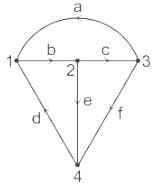• In the above graph, the direction of the current flow is represented with an arrow in each branch. Hence, it is an oriented graph.
Test: Graph Theory - 2 - Question 5

If one link fails, the entire network can be disabled in ______.

Detailed Solution for Test: Graph Theory - 2 - Question 5

Concept:

A single cable links all of the included nodes in a bus topology. The primary cable serves as the network's backbone. If the common cable breaks, the entire system will be brought to a halt.

Every device in a ring network has exactly two neighbors for communication purposes. It's called ring topology because it's shaped like a ring. Every computer in this topology is linked to another computer. A single computer failure can disrupt the entire network.

As a result, bus and ring topologies have difficulties, such as the possibility of the entire network being deactivated if one connection fails.

Hence the correct answer is Bus and Ring Topology.

Test: Graph Theory - 2 - Question 6

To construct the dual of a four-mesh network how many nodes are required?

Detailed Solution for Test: Graph Theory - 2 - Question 6

Concept:

Duality:

• Two electrical networks are said to be dual networks if the mesh equations of one network are equal to the node equation of others.
• Identical behavior patterns observed between voltages and currents in two circuits illustrate the principle of duality.
• The dual networks are based on Kirchhoff Current Law and Kirchhoff Voltage Law.

Nodal Analysis:

Nodal analysis is a method of analyzing networks with the help of KCL equations.

For a network of N nodes, the number of simultaneous equations to be solved to get the unknowns

= Number of KCL equations

= N - 1

Mesh Analysis:

Mesh analysis is a method of analyzing networks with the help of KVL equations.

For a network having N nodes and B branches, the number of simultaneous equations to be solved to get the unknowns

= Number of KVL equations

= number of independent loop equations

= B - N + 1

Application:

Given: KVL equations = 4,

Therefore, B - N + 1 = 4

B = 3 + N ........(1)

For duality of two networks,

Mesh equations of one network = nodal equations of other

Hence,

B - N + 1 = N - 1

N = B + 2 / 2 .....(2)

substitute (1) in (2)

N = N + 5 / 2

N = 5

Test: Graph Theory - 2 - Question 7

The number of twigs and links in a connected network graph with 'n' nodes and 'b' branches are, respectively.

Detailed Solution for Test: Graph Theory - 2 - Question 7

Concept:

Tree: A tree is a sub graph of main graph which connects all the nodes without forming a closed loop.

For a graph with ‘n’ nodes, the rank of tree = n – 1

Any tree for a given graph can be constructed with (n–1) branches.

Twig: The branch of a tree is called as twig indicated by thick Line. Any tree with n nodes has (n – 1) twigs.

Co-tree: The set of branches in a graph other than tree branches form a co tree.

Link: The branch of a co tree is called link indicated by dotted Line.

For any graph with n nodes and b branches, the numbers of links is given by:

= b – n + 1

Test: Graph Theory - 2 - Question 8

Considering the principle of duality, which of the following pair is INVALID dual pair?

Detailed Solution for Test: Graph Theory - 2 - Question 8

Duality:

• Two electrical networks are said to be dual networks if the mesh equations of one network are equal to the node equation of others.
• Identical behavior patterns observed between voltages and currents in two circuits illustrate the principle of duality.
• The dual networks are based on Kirchhoff Current Law and Kirchhoff Voltage Law.

Some important dual relations are given below.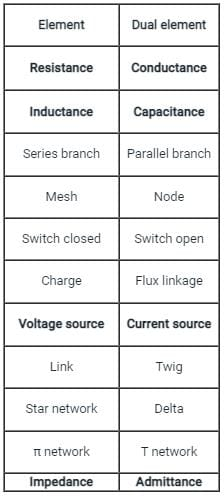Test: Graph Theory - 2 - Question 9

A loop which does not contain any other loop within it is called _________.

Detailed Solution for Test: Graph Theory - 2 - Question 9

Node:

A point or junction where two or more circuit elements (resistor, capacitor, inductor, etc.) meet is called Node. In other words, a point of connection between two or more branches is known as a Node.

Supernode:

If a voltage source is connected between two non-reference nodes, then we combine the two nodes as to yield super node.

Branch:

That part or section of a circuit locate between two junctions is called the branch. In a branch, one or more elements can be connected, and they have two terminals.

Loop:

A closed path in a circuit where more than two meshes can occur is known as Loop i.e. there may be many meshes in a loop, but a mesh does not contain on one loop.

Mesh:

A closed-loop that contains no other loop within it or a path which does not contain other paths is called Mesh.

Super mesh:

If a current source is present at the common boundary of two meshes, then we create a super mesh by avoiding the current source and any element connected to it in series.

Note: The number of independent loops for a network with n nodes and b branches is = b - n + 1

Test: Graph Theory - 2 - Question 10

The number of closed paths in a tree of the graph is:

Detailed Solution for Test: Graph Theory - 2 - Question 10

Tree: A tree is a connected subgraph of a connected graph containing all the nodes of the graph but containing no loops, i.e., there is a unique path between every pair of nodes.

Therefore, the number of closed paths in a tree of the graph is zero.

Twig: The branches of the tree are called twigs.

Link: Those branches of the graph which are not in the tree.

Co-tree: All the links of a tree together constitute complement of the tree and is called co-tree, in which the number of branches is equal to b - (n - 1)

Where b is the number of branches of the graph.

Number of twigs: t = n - 1

Number of links: L = b - t = b – n + 1

Test: Graph Theory - 2 - Question 11

Consider the following data for twigs and links:

N = Number of nodes

L = Total number of links

B = Total number of branches

The total number of links associated with a tree is

Detailed Solution for Test: Graph Theory - 2 - Question 11

Concept:

Tree: A tree is a sub graph of main graph which connects all the nodes without forming a closed loop.

For a graph with ‘n’ nodes, the rank of tree = N – 1

Any tree for a given graph can be constructed with (N–1) branches.

Twig: The branch of a tree is called as twig indicated by thick Line. Any tree with n nodes has (n–1) twigs.

Co-tree: The set of branches in a graph other than tree branches form a co tree.

Link: The branch of a co tree is called link indicated by dotted Line.

For any graph with n nodes and b branches, the numbers of links is given by:

= B – N + 1

Test: Graph Theory - 2 - Question 12

The graph of a network has 8 nodes and 5 independent loops. The number of branches of the graph is

Detailed Solution for Test: Graph Theory - 2 - Question 12

Concept:

Nodal Analysis:

Nodal analysis is a method of analyzing networks with the help of KCL equations.

For a network of N nodes, the number of simultaneous equations to be solved to get the unknowns

= Number of KCL equations

= N - 1

Mesh Analysis:

Mesh analysis is a method of analyzing networks with the help of KVL equations.

For a network having N nodes and B branches, the number of simultaneous equations to be solved to get the unknowns

= Number of KVL equations

= number of independent loop equations

= B - N + 1

Where, B = no of the branch, N = No of node

Calculation:

Given that,

Number of nodes (N) = 8

Number of independent loops (L) = 5

Let the number of branches are B.

We know that,

L = B – N + 1

⇒ 5 = B – 8 + 1 ⇒ B = 12

Test: Graph Theory - 2 - Question 13

Consider the following statements regarding trees:

1. A tree contains all the nodes of the graph.

2. A tree shall contain any one of the loops.

3. Every connected graph has at least one tree.

Which of the above statements are correct?

Detailed Solution for Test: Graph Theory - 2 - Question 13

Let us consider the following electric circuit: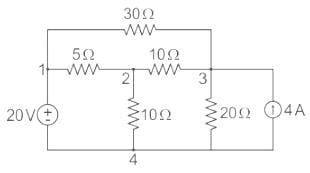An equivalent graph corresponding to the above electric circuit is shown in the following figure: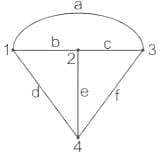Tree:

The tree is a connected subgraph of a given graph, which contains all the nodes of a graph. But, there should not be any loop in that subgraph. The branches of a tree are called as twigs.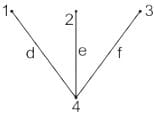This connected subgraph contains all the four nodes of the given graph and there is no loop.

Hence, it is a Tree.

Test: Graph Theory - 2 - Question 14

Consider the following with regards to graph as shown in the figure given below: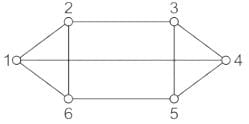1. Regular graph

2. Connected graph

3. Complete graph

4. Non-regular graph

Which of the above are correct?

Detailed Solution for Test: Graph Theory - 2 - Question 14

Concept:

• A graph is said to be a regular graph if all nodes connected with other nodes in the same manner, otherwise the graph will be non-regular.
• A graph is said to be a connected graph, if at least one branch must present between any two nodes of the graph otherwise it will be an unconnected graph.
• A graph is said to be a complete graph if every node in the graph is connected with every other node in the graph.

Analysis:

A network graph is given as:From the network graph above, we have:

Total nodes or vertex = 6

= (1, 2, 3, 4, 5, 6)

And Total edges or branches = 9

= (1 - 2), (2 - 3), (3 - 4), (4 - 5), (5 - 6), (6 - 1), (1 - 4), (2 - 6), (3 - 5)

• Since each node of the graph is connected via three other nodes. So it is a regular graph.
• i.e. for Node 1: Node 2, 4, 6 are connected from node-1
• for node-2: nodes 1, 6, 3 are connected from node 2, and so on.
• In the network graph, a defined path exists between every pair of nodes. So it is a connected graph.
• Since in the given network graph, every node is not connected with every other node. So it is not a complete graph.
• e.g. node 1 is not connected from node 3, 5
• node 2 is not connected from nodes 4, 5, and so on.
Test: Graph Theory - 2 - Question 15

What are the properties of a tree in a network graph?

1. It consists of all the nodes of the graph.

2. If the graph has N number of nodes, the tree will have (N – 1) branches.

3. There will be only one closed path in the tree.

Detailed Solution for Test: Graph Theory - 2 - Question 15

Tree: A tree is a subgraph of the main graph which connects all the nodes without forming a closed loop.

For a graph with ‘n’ nodes, the rank of tree = n – 1

Any tree for a given graph can be constructed with (n–1) branches.

Twig: The branch of a tree is called twig indicated by a thick line. Any tree with n nodes has (n–1) twigs.

Co-tree: The set of branches in a graph other than tree branches form a co tree.

Link: The branch of a co tree is called the link indicated by the dotted line. For any graph with n nodes and b branches, numbers of links = b – n + 1

Example:

A network with 4 nodes and corresponding graphical representation is represented below: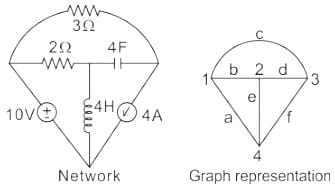Now, if we remove the branches c, a, and f from the circuit, we will get the tree as shown below: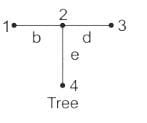We observe that the tree for the given network contains all the four nodes of the network but does not form any closed path.  (Statement 1 is correct, but Statement 3 is incorrect)

We can also observe that there are 3 twigs or branches of a tree in the given electric network. The number of nodes in the network is 4.

∴ The number of branches/twigs = Number of nodes - 1. (Statement 2 is correct)

## Network Theory (Electric Circuits)

23 videos|63 docs|60 tests
 Use Code STAYHOME200 and get INR 200 additional OFF Use Coupon Code
Information about Test: Graph Theory - 2 Page
In this test you can find the Exam questions for Test: Graph Theory - 2 solved & explained in the simplest way possible. Besides giving Questions and answers for Test: Graph Theory - 2, EduRev gives you an ample number of Online tests for practice

## Network Theory (Electric Circuits)

23 videos|63 docs|60 tests